Algebra

Equations

Inequalities

Graphs

Numbers

Calculus

Matrices

Tutorials

# Addition and Subtraction of Polynomials

The sum of two speciﬁc numbers can be written as a third speciﬁc number. Given the speciﬁc numbers 2 and 3, we can write their sum as 2+3, or as 5.

The sum of the literal numbers a and b can merely be indicated as a + b. The a and b are called terms of the sum.

Terms that have identical literal factors are called like terms2 abc, 3 bac, -10 cba are like terms. On the other hand, 2 abc and 3 abd are unlike terms, since 2 abc has c as a factor while 3 abd does not. The numerical coefﬁcients of the terms do not affect whether the terms are like or unlike.

When the terms to be added are like terms, such as 5 a and 7 a, the sum 5 a+7 a can be simpliﬁed by use of the distributive law of multiplication.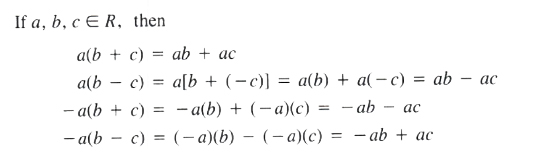Using the distributive law of multiplication, we have

5 a+7 a = (5+7) a = 12 a

Also 4 a+a-8 a = (4+1) a-8 a

=5 a-8 a

=(5-8) a

=- 3 a

or 4 a+a-8 a = (4+1-8) a

= 3 a

It is important to realize that

2 a+4 a = (2+4) a = 6 a

and 5 a-3 a = (5-3) a = 2 a (not just 2)

When we add polynomials, we combine only like terms in the polynomials

Let's see how our Polynomial solver simplifies this and similar problems. Click on "Solve Similar" button to see more examples solved step by step.

Example Add 3 a-5 b and  -2 a+2 b

Solution (3 a-5 b)+( 2 a+3 b) = 3 a-5 b-2 a+3 b

=(3 a-2 a)+( 5 b+3 b)

=(3-2) a+( 5+3) b = a-2 b

Example Add 3 a-2 b+c and 6 a+4 b-5 c

Solution (3 a+2 b+c)+(6 a+4 b-5 c) = 3 a-2 b+c+6 a+4 b-5 c

=(3 a+6 a)+( 2 b+4 b)+(c-5 c)

=(3+6) a+( 2+4) b+(1-5) c

=9 a+2 b-4 c

An easy way to ﬁnd the sum of polynomials is to write the polynomials in rows one under the other, so that like terms are in the same column. This is similar to addition of

Speciﬁc numbers, when we write speciﬁc numbers in rows so that the units. tens, hundreds, and so forth are in separate columns.

Example Find the sum of the following polynomials

2 ab-6 c+d 3 c-5 d and 2 d-4 ab+4 c

Solution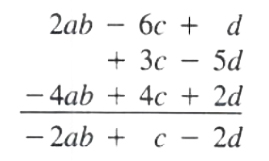(2 ab-6 c+d)+(3 c-5 d)+(2 d-4 ab+4 c)= 2 ab+c-2 d

Let's see how our Polynomial solver shows all the solution steps for this and similar problems. Click on "Solve Similar" button to see more examples.

Subtraction of Polynomials

In algebraic language we symbolize the subtraction of b from a by a-b, which is the same as a+( b). That is. to subtract b from a, we add the additive inverse (the negative) of b to a.

The additive inverse of +6 x is -6 x. That is, -(+ 6 x) = -6 x.

The additive inverse of - 10 y is + 10 y. That is, -( 10 y) = +10 y

When the terms to be subtracted are like terms. the difference can be simpliﬁed using the distributive law of multiplication.

Example 1. Subtract (3 a) from(8 a)

(8 a)-(3 a) = 8 a-3 a = (8-3) a = 5 a

2. Subtract ( 3 a) from (8 a).

(8 a)-( 3 a) = 8 a+3 a = (8+3) a = 11 a

3. Subtract (3 a) from ( 8 a).

( 8 a)-(3 a) = 8 a-3 a = ( 8-3) a = 11 a

4. Subtract ( 3 a) from ( 8 a).

( 8 a)-( 3 a) = 8 a+3 a = ( 8+3) a = 5 a

To subtract one polynomial, called the subtrahend, from another polynomial, called the minuend, add the minuend to the additive inverse of the subtrahend and combine like terms.

The additive inverse of a polynomial is the additive inverse of every term in the polynomial.

Subtraction of Polynomials

The additive inverse of 5 a-6 b+8 is 5 a+6 b-8 That is,

(5 a-6 b+8) = 5 a+6 b-8

Example Subtract (3 a-5 b) from (6 a-7 b)

Solution (6 a-7 b)-(3 a-5 b) = 6 a-7 b-3 a+5 b

=(6 a-3 a)+( 7 b+5 b)

=(6-3) a+( 7+5) b

=3 a-2 b

Let's see how our polynomial solver simplifies this problem step by step. Click on "Solve Similar" button to see more examples.

Example Subtract (3 a-2 b+5) from (8 a+6 b-2)

Solution (8 a+6 b-2) (3 a-2 b+5) = 8 a+6 b-2-3 a+2 b-5

=(8 a-3 a)+(6 b+2 b)+( 2-5)

=(8-3) a+(6+2) b+( 2-5)

=5 a+8 b-7

We can subtract polynomials more simply by writing them in rows. Write the minuend in the ﬁrst row and the subtrahend in the second row, so that like terms are in the same column. The additive inverse of a polynomial is the same polynomial with the signs of the terms changed. Thus we change the signs of each term in the subtrahend. write the new signs in circles above the original signs, and add like terms using the new signs.

Example From 7 ab-2 c+8 subtract 8 ab-5 c+4

Solution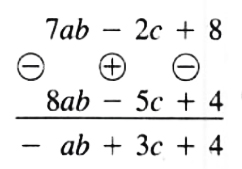(7 ab-2 c+8)-(8 ab-5 c+4) = ab+3 c+4

Let's see how our Polynomial simplifier shows step by step solutions to  this and similar problems. Click on "Solve Similar" button to see more examples.

Example Subtract 2 x-3 y-6 from 4 x-3 y+10

Solution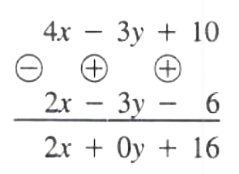Since 0 , y=0, that term does have to be written.

(4 x-3 y+10) - (2 x-3 y-6) = 2 x+16

Example Subtract 6 ab+2 c-4 from 8 ab-2 b+3

Solution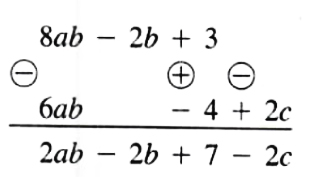(8 ab-2 b+3)-(6 ab+2 c-4) = 2 ab-2 b+7-2 c

 ← Previous Tutorial Next Tutorial →